• 上一节介绍了Lazarus一般的开发操作流程，对于不熟悉pascal语言的朋友可能看的还是不大明白，不知道pascal代码里都应该包含什么或起什么作用，这回就简单地介绍下语法及代码文件的结构。当然，只是描述一下通常会...

上一节介绍了Lazarus一般的开发操作流程，对于不熟悉pascal语言的朋友可能看的还是不大明白，不知道pascal代码里都应该包含什么或起什么作用，这回就简单地介绍下语法及代码文件的结构。当然，只是描述一下通常会用到的东西，如果想深入了解pascal，请参考本节最后推荐的书。

free pascal的代码文件一般只有两种，.lpr和.pas（或.pp）；lpr文件是工程文件，pas或pp是单元文件。一个完整的pascal工程必须包括一个lpr文件。来看一下Lazarus默认创建的工程都包括些什么。打开Lazarus后点击全部保存，选择路径并确定，保存为默认的文件名。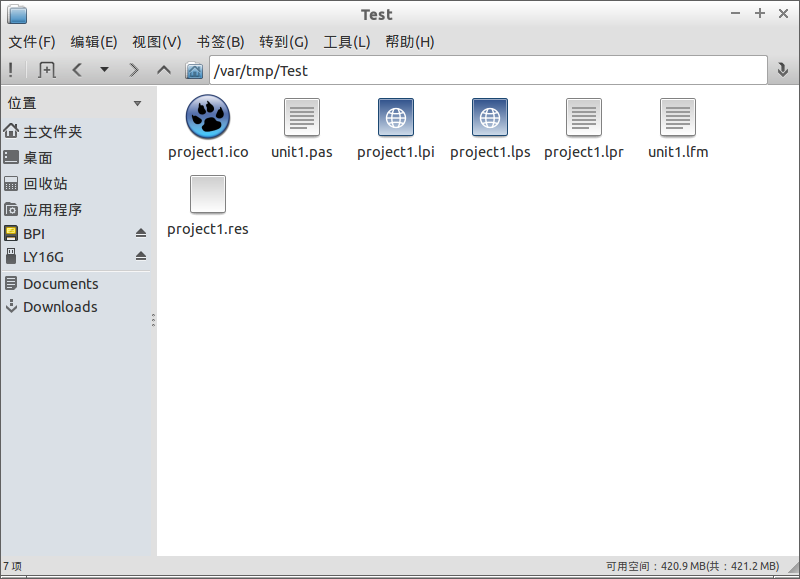project1.lpr - 工程文件，整个程序的入口，一般情况下不需要手动修改；

project1.res - 工程资源文件，保存版本、字符串等，不需要手动修改；

project1.lpi - 工程管理文件，保存工程的信息，Lazarus靠此文件管理所有的文件为一个工程，不需要手动修改；

project1.lps - IDE环境关闭时的信息，包括各种编辑窗体最后的位置等信息，不需要手动修改；

project1.ico - 图标文件，编译为可执行文件后显示的图标，不需要手动修改；

unit1.pas - 窗体源代码文件，可手动添加代码；

unit1.lfm - 窗体属性代码文件，不需要手动修改；

可以看到，真正需要编写代码的地方只有一个文件，unit1.pas。后面我们就着重分析一下这个文件的结构。

注：unit文件保存的时候也可以选择扩展名为.pp。.pp和.pas文件的关系：.pp就是.pas文件。Delphi默认的源代码文件为.pas，而Lazarus的源代码文件为.pp。Lazarus为了兼容Delphi，可以支持这两种扩展名，所以除了扩展名不同意外它们没有任何区别。

下面是一个pas文件的代码，我做了些注释，可以很清晰地看出各个部分的作用。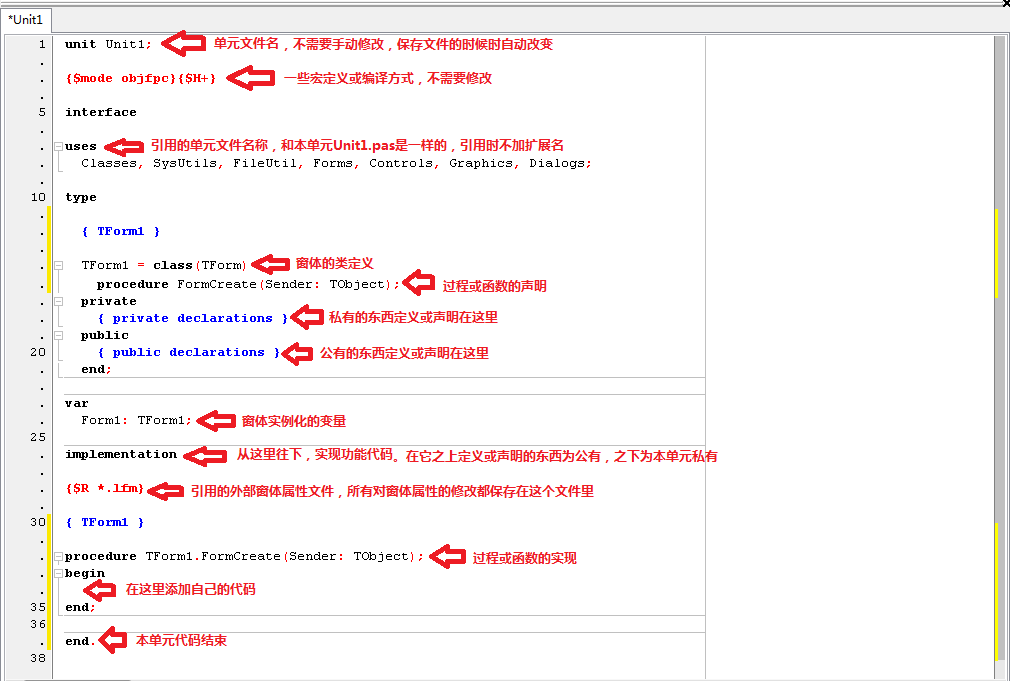pascal的代码文件不分.h或.c，都包含在了.pas文件中，使文件结构更加简洁。需要对外开放的函数或定义，只要写在implementation之上就可以了，代码编辑器在写入.后会自动提示可见的东西，不可见的则不会被显示。

结构说的差不多了，还是来看看pascal的语法吧。语法和C语言大同小异，只是把C里的{、}换成了begin、end，变量定义的名称和类型调换一下位置就算是会pascal了。呵呵，这样说法未免太不负责任，会遭到鄙视的。不过在语法上真的差别不大，差别最大的地方是pascal有类这个东西。而类的说明我希望留在下一节，结合ClassA20的类库来讲解，这样更容易接受。本节还是继续基础的东

西吧。

pascal不区分大小写，以下为本人的习惯写法，仅供参考。

1.数据类型：

C

pascal

int

Integer

short

ShortInt

long

LongInt

unsigned char

Byte

unsigned short

Word

unsigned long

LongWord或DWORD

float

Single

double

Double或Real

Boolean

*p

Pointer

&p

@p或Pointer(p);

想起来多少写多少，也许有错误，欢迎补充和指正。

2.操作符：

C

pascal

=

:=

+

+

-

-

*

*

/

div

%

mod

<<

shl

>>

shr

&

and

|

or

!

not

^

xor

想起来多少写多少，也许有错误，欢迎补充和指正。

3.循环语句：

c

pascal

for (i = 0; i < 10; i++)

for i:=0 to 9 do

while (1) ...;

while (1) do ...;

do ... while (1);

repeat ... until (1);

想起来多少写多少，也许有错误，欢迎补充和指正。

4.比较语句：

c

pascal //有没有括号都可以

if (A == B) ... ;

if A = B then ... ;

if (A || B) ... ;

if A or B then ... ;

if (A && B) ... ;

if A and B then ...;

switch (A)
{
case 1:
break;
case 2:
break;
default:
break;
}

case A of
1: ;
2: ;
else
end;

想起来多少写多少，也许有错误，欢迎补充和指正。

5.函数定义：

c

pascal

void fun();

procedure fun;

int fun();

function fun:Integer;

想起来多少写多少，也许有错误，欢迎补充和指正。

6.着重说一下String：

C中没有字符串这个类型，只有字符数组char[]，以'\0'结束。

pascal对字符串操作要强大很多，可以定义数组array of Char或array of Byte;

还有一个String类型。String即可以当错数组使用，也可以当成字符串使用，可以随时改变长度SetLength(String, 10)，并且不需要手动释放当所在的函数生命周期结束后也就随之自动释放了(结构体中除外)。

数组转字符串：String(Byte数组或Char数组)

字符串转数组：String = @Byte数组;

取下标：Char:= String; 或 Byte:= Ord(String);

字符串相加：String:= String1 + String2;

字符串插入：Insert(String, SubString, Index);

字符串删除：Delete(String, Index, Count);

...

所以我一般在程序中都以String类型作为Buffer来进行传输或处理，操作很方便。

想起来多少写多少，也许有错误，欢迎补充和指正。

7.着重说一下异常处理：

C中没有异常处理。

pascal有try，try有两种：

try

//执行的代码部分

except

//异常处理部分

end;

当执行代码部分发生了任何执行失败，使用try可以不至于使整个程序崩溃停止运行，而是可以跳转到异常处理部分进行错误处理，或可以友好地提示用户。

try

//执行代码部分

finally

//强制执行部分

end;

无论在代码执行部分执行任何的打断或退出等语句，finally部分都会被执行到，一般用于释放资源或其他必要的处理。

两者可以结合使用：

try

try

//代码

except

//异常处理

end;

//代码

finally

//强制执行

end;

常用的东西基本上就是这些，下一节开始正式讲解ClassA20类库，敬请期待。

如果想下载ClassA20的封装类库文件，请访问：https://github.com/tjCFeng/ClassA20。


展开全文delphi lazarus arm linux
• 什么是语法解析？ 在自然语言学习过程中，每个人一定都学...在自然语言的处理过程中，有许多应用场景都需要考虑句子的语法，因此研究语法解析变得非常重要。 语法解析有两个主要的问题，其一是句子语法在计算机中的


原文：http://blog.csdn.net/lanxu_yy/article/details/37700841

什么是语法解析？
在自然语言学习过程中，每个人一定都学过语法，例如句子可以用主语、谓语、宾语来表示。在自然语言的处理过程中，有许多应用场景都需要考虑句子的语法，因此研究语法解析变得非常重要。

语法解析有两个主要的问题，其一是句子语法在计算机中的表达与存储方法，以及语料数据集；其二是语法解析的算法。

对于第一个问题，我们可以用树状结构图来表示，如下图所示，S表示句子；NP、VP、PP是名词、动词、介词短语（短语级别）；N、V、P分别是名词、动词、介词。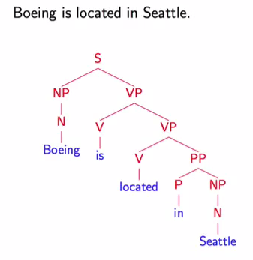实际存储的时候上述的树可以表示为(S (NP (N Boeing)) (VP (V is) (VP (V located) (PP (P in) (NP (N Seattle))))))。互联网上已经有成熟的、手工标注的语料数据集，例如The Penn Treebank Project （Penn
Treebank II Constituent Tags）。

对于第二个问题，我们需要有合适的算法来处理。这也是我们本章将要讨论的内容。

上下文无关语法（Context-Free Grammer）
为了生成句子的语法树，我们可以定义如下的一套上下文无关语法。
1）N表示一组非叶子节点的标注，例如{S、NP、VP、N...}
2）Σ表示一组叶子结点的标注，例如{boeing、is...}
3）R表示一组规则，每条规则可以表示为X->Y1Y2...Yn，X∈N，Yi∈(N∪Σ)
4）S表示语法树开始的标注

举例来说，语法的一个语法子集可以表示为下图所示。当给定一个句子时，我们便可以按照从左到右的顺序来解析语法。例如，句子the man sleeps就可以表示为(S (NP (DT the) (NN man)) (VP sleeps))。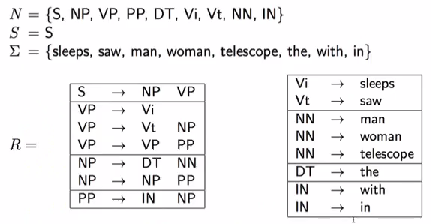这种上下文无关的语法可以很容易的推导出一个句子的语法结构，但是缺点是推导出的结构可能存在二义性。例如下面两张图中的语法树都可以表示同一个句子。常见的二义性问题有：1）单词的不同词性，如can一般表示“可以”这个情态动词，有时表示罐子；2）介词短语的作用范围，如VP PP PP这样的结构，第二个介词短语可能形容VP，也可能形容第一个PP；3）连续的名字，如NN NN NN。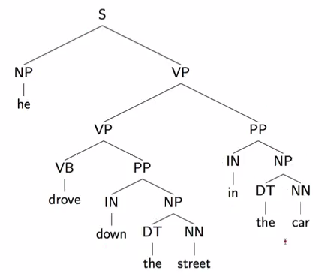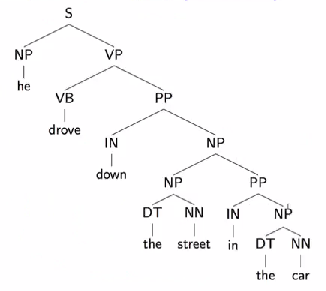概率分布的上下文无关语法（Probabilistic Context-Free Grammar）
由于语法的解析存在二义性，我们就需要找到一种方法从多种可能的语法树种找出最可能的一棵树。一种常见的方法既是PCFG （Probabilistic Context-Free Grammar）。如下图所示，除了常规的语法规则以外，我们还对每一条规则赋予了一个概率。对于每一棵生成的语法树，我们将其中所以规则的概率的乘积作为语法树的出现概率。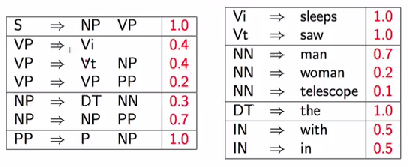综上所述，当我们或得多颗语法树时，我们可以分别计算每颗语法树的概率p(t)，出现概率最大的那颗语法树就是我们希望得到的结果，即arg max p(t)。

训练算法
我们已经定义了语法解析的算法，而这个算法依赖于CFG中对于N、Σ、R、S的定义以及PCFG中的p(x)。上文中我们提到了Penn Treebank通过手工的方法已经提供了一个非常大的语料数据集，我们的任务就是从语料库中训练出PCFG所需要的参数。
1）统计出语料库中所有的N与Σ；
2）利用语料库中的所有规则作为R；
3）针对每个规则A -> B，从语料库中估算p(x) = p(A -> B) / p(A)；

在CFG的定义的基础上，我们重新定义一种叫Chomsky的语法格式。这种格式要求每条规则只能是X -> Y1 Y2或者X -> Y的格式。实际上Chomsky语法格式保证生产的语法树总是二叉树的格式，同时任意一棵语法树总是能够转化成Chomsky语法格式。

语法树预测算法
假设我们已经有一个PCFG的模型，包含N、Σ、R、S、p(x)等参数，并且语法树总数Chomsky语法格式。当输入一个句子x1, x2, ... , xn时，我们要如何计算句子对应的语法树呢？
第一种方法是暴力遍历的方法，每个单词x可能有m = len(N)种取值，句子长度是n，每种情况至少存在n个规则，所以在时间复杂度O(m*n*n)的情况下，我们可以判断出所有可能的语法树并计算出最佳的那个。
第二种方法当然是动态规划，我们定义w[i, j, X]是第i个单词至第j个单词由标注X来表示的最大概率。直观来讲，例如xi, xi+1, ... , xj，当X=PP时，子树可能是多种解释方式，如(P NP)或者(PP PP)，但是w[i, j, PP]代表的是继续往上一层递归时，我们只选择当前概率最大的组合方式。特殊情况下，w[i, i, X] = p(X -> xi)。因此，动态规划的方程可以表示为w[i, j, X] = max (p(X -> Y Z) * w(i, s, Y) * w(s+1,
j, Z))。关于动态规划方法，leetcode里有不少案例可以说明。

语法解析按照上述的算法过程便完成了。虽说PCFG也有一些缺点，例如：1）缺乏词法信息；2）连续短语（如名词、介词）的处理等。但总体来讲它给语法解析提供了一种非常有效的实现方法。


展开全文NLP PCFG
• ## 语法基础——C++语法基础

千次阅读 多人点赞 2017-08-31 22:23:18
前言 最近发现要学习C++来开发NDK，不得不把基础的东西记录下来，否则学的太多会混淆，废话不多说，开始记录我的C++学习之旅吧 HelloWord 导库 命名空间 输出函数 #include &...Hel...
前言

最近发现要学习C++来开发NDK，不得不把基础的东西记录下来，否则学的太多会混淆，废话不多说，开始记录我的C++学习之旅吧

HelloWord

导库
命名空间
输出函数
#include <iostream>
//必须带有命名空间才能使用cout等
using namespace std;
int main()
{
cout << "Hello, world!" << endl;
return 0;
}

命名空间

1、命名空间属性访问和结构体访问

namespace NSP_A{
int a = 9;
struct Student{
char name;
int age;
};
}

void main(){
//使用命名空间
cout << NSP_A::a << endl;

//使用命名空间中的结构体
using NSP_A::Student;
Student t;
}

2、命名空间的嵌套

namespace NSP_B{
//命名空间嵌套
namespace NSP_C{
int c = 90;
}
}

void main(){
cout << NSP_B::NSP_C::c << endl;
}

类

1、类、属性、方法的声明与使用

#define _CRT_SECURE_NO_WARNINGS
#include <stdlib.h>
#include <iostream>
#include <stdarg.h>
using namespace std;
#define PI 3.14
class MyCircle{
//属性（共用权限访问修饰符）
private:
double r;
double s;
public:
void setR(double r){
this->r = r;
}
//获取面积
double getS(){
return PI * r * r;
}
};

void main(){
MyCircle c;
c.setR(4);

cout << "圆的面积：" << c.getS() << endl;
system("pause");
}

2、类的大小

类的大小只计算普通属性的大小，其他都不包括在内

class A{
public:
int i;
int j;
int k;
static int m;
};
class B{
public:
int i;
int j;
int k;
void myprintf(){
cout << "打印" << endl;
}
};
void main(){
//输出的结果都是12
cout << sizeof(A) << endl;
cout << sizeof(B) << endl;
}

继承

1、继承基本使用

class Human{
public:
void say(){
cout << "说话" << endl;
}
protected:
int age;
};
class Man : public Human{

};

void main(){
//子类
Man m1;
m1.say();
//将子类赋值给父类的引用或指针
Human* h_p = &m1;
h_p->say();
Human &h1 = m1;
h1.say();
//子类对象初始化父类类型的对象
Human h2 = m1;
h2.say();
//子类对象调用父类的成员
m1.Human::say();
m1.Human::age = 10;
}

2、向父类构造方法传参

class Human{
public:
Human(char* name, int age){
this->name = name;
this->age = age;
}
protected:
char* name;
int age;
};
class Man : public Human{
public:
//给父类构造函数传参，同时给属性h对象赋值
Man(char *brother, char *s_name, int s_age, char *h_name, int h_age) : Human(s_name, s_age), h(h_name,h_age){
this->brother = brother;
}
private:
Human h;
};

void main(){
Man m1("Hensen","HensenBoy",18,"HensenGirl",18);
}

3、多继承的实现

class Person{

};

class Citizen{

};

class Student : public Person, public Citizen{

};

4、继承间的访问修饰符

基类中        继承方式        子类中
public     ＆ public继承 = > public
public     ＆ protected继承 = > protected
public     ＆ private继承 = > private

protected  ＆ public继承 = > protected
protected  ＆ protected继承 = > protected
protected  ＆ private继承 = > private

private    ＆ public继承 = > 子类无权访问
private    ＆ protected继承 = > 子类无权访问
private    ＆ private继承 = > 子类无权访问

5、继承的二义性

virtual：表示虚继承，不同路径继承来的同名成员只有一份拷贝，解决不明确的问题

class A{
public:
char* name;
};
//这里面加了virtual关键字
class A1 : virtual public A{

};
//这里面加了virtual关键字
class A2 : virtual public A{

};
class B : public A1, public A2{

};

void main(){
B b;
//如果程序不加virtual关键字就会导致二义性，系统无法辨识哪个类的name属性，会报错
b.name = "Hensen";
//这里通过指定父类显示调用是可以的
b.A1::name = "Hensen";
b.A2::name = "Hensen";
}

多态

1、多态的基本使用

//Plane.h文件
#pragma once
//普通飞机
class Plane{
public:
virtual void fly();
virtual void land();
};
//Plane.cpp文件
#include "Plane.h"
#include <iostream>
using namespace std;
void Plane::fly(){
cout << "起飞" << endl;
}
void Plane::land(){
cout << "着陆" << endl;
}

//Jet.h文件
#pragma once
#include "Plane.h"
//直升飞机
class Jet : public Plane{
virtual void fly();
virtual void land();
};
//Jet.cpp文件
#include "Jet.h"
#include <iostream>
using namespace std;
void Jet::fly(){
cout << "直升飞机在原地起飞..." << endl;
}
void Jet::land(){
cout << "直升飞机降落在女神的屋顶..." << endl;
}

#include "Plane.h"
#include "Jet.h"
#include "Copter.h"
//业务函数
void bizPlay(Plane& p){
p.fly();
p.land();
}
void main(){
Plane p1;
bizPlay(p1);
//直升飞机
Jet p2;
bizPlay(p2);
}

引用

1、引用的使用

void main(){
int a = 10;
//&是C++中的引用，引用：变量的另外一个别名，共用同个地址
int &b = a;
cout << b << endl;
}

2、引用与指针的区别

不存在空引用，引用必须连接到一块合法的内存。
一旦引用被初始化为一个对象，就不能被指向到另一个对象。指针可以在任何时候指向到另一个对象。
引用必须在创建时被初始化。指针可以在任何时间被初始化。
3、引用与指针写法上的差异

struct Teacher{
char* name;
int age;
};
//带有结构体指针的写法
void myprint(Teacher *t){
cout << t->name << "," << t->age << endl;
//(*t).name
}
//带有结构体引用的写法
void myprint2(Teacher &t){
cout << t.name << "," << t.age << endl;
t.age = 21;
}
//指针值交换
void swap_1(int *a, int *b){
int c = 0;
c = *a;
*a = *b;
*b = c;
}
//引用值交换
void swap_2(int &a, int &b){
int c = 0;
c = a;
a = b;
b = c;
}
void main(){
Teacher t;
t.name = "Hensen";
t.age = 20;
//指针的写法
myprint(&t);
//引用的写法
myprint2(t);

int x = 10;
int y = 20;
//指针的写法
swap_1(&x, &y);
//引用的写法
swap_2(x,y);
}

4、引用的作用

把引用作为参数：C++支持把引用作为参数传给函数，这比传一般的参数更安全
把引用作为返回值：可以从C++函数中返回引用，就像返回其他数据类型一样
5、指针引用，代替二级指针

struct Teacher{
char* name;
int age;
};
//引用的写法
void getTeacher(Teacher* &p){
p = (Teacher*)malloc(sizeof(Teacher));
p->age = 20;
}
//二级指针的写法，原本应该这样写，但是已经被引用的写法代替了
void getTeacher(Teacher **p){
Teacher *tmp = (Teacher*)malloc(sizeof(Teacher));
tmp->age = 20;
*p = tmp;
}

void main(){
Teacher *t = NULL;
//传递引用的指针t，相当于二级指针
getTeacher(&t);
}

6、常引用，类似于java中final

//常引用在方法中的引用
void myprint(const int &a){
cout << a << endl;
}

void main(){
//引用必须要有值，不能为空，下面写法是错误的
//const int a;
//int &a = NULL;

//常引用属性使用一
int a = 10, b = 9;
const int &c = a;
//常引用属性使用二
const int &d = 70;
}

7、引用与指针的大小

struct Teacher{
char name;
int age;
};

void main(){
Teacher t;
//引用
Teacher &t1 = t;
//指针
Teacher *p = &t;

//结果是24，引用相当于变量的别名
cout << sizeof(t1) << endl;
//结果是4，指针只是存放的地址
cout << sizeof(p) << endl;
system("pause");
}

异常

1、C++异常处理，会根据抛出的异常数据类型，进入到相应的catch块中

void main(){
try{
int age = 300;
if (age > 200){
throw 9.8;
}
}
catch (int a){
cout << "int异常" << endl;
}
catch (char* b){
cout << b << endl;
}
catch (...){
cout << "未知异常" << endl;
}
}

2、向下抛出异常

void mydiv(int a, int b){
if (b == 0){
throw "除数为零";
}
}
void func(){
try{
mydiv(8, 0);
}
catch (char* a){
throw a;
}
}
void main(){
try{
func();
}
catch (char* a){
cout << a << endl;
}
}

3、抛出对象

class MyException{

};
void mydiv(int a, int b){
if (b == 0){
throw MyException();
//不要抛出异常指针
//throw new MyException;
}
}
void main(){
try{
mydiv(8,0);
}
catch (MyException& e2){
cout << "MyException引用" << endl;
}
catch (MyException* e1){
cout << "MyException指针" << endl;
//指针的话需要手动释放内存
delete e1;
}
}

4、方法抛出异常

void mydiv(int a, int b) throw (char*, int) {
if (b == 0){
throw "除数为零";
}
}

5、标准异常

//需要导入标准异常的依赖库
#include<stdexcept>

class NullPointerException : public exception{
public:
NullPointerException(char* msg) : exception(msg){

}
};
void mydiv(int a, int b){
if (b > 10){
throw out_of_range("超出范围");
}
else if (b == NULL){
throw NullPointerException("为空");
}
else if (b == 0){
throw invalid_argument("参数不合法");
}
}
void main(){
try{
mydiv(8,NULL);
}
catch (out_of_range e1){
cout << e1.what() << endl;
}
catch (NullPointerException& e2){
cout << e2.what() << endl;
}
catch (...){
cout << "未知异常" << endl;
}
}

6、外部类异常

class Err{
public:
class MyException{
public:MyException(){

}
};
};
void mydiv(int a, int b){
if (b > 10){
throw Err::MyException();
}
}

IO流

1、普通文件

#include <iostream>
#include <fstream>
void main(){
char* fname = "c://dest.txt";
//输出流
ofstream fout(fname);
//创建失败
return;
}
//写入文件
fout << "Hensen" << endl;
//关闭输出流
fout.close();

//输入流
ifstream fin(fname);
//创建失败
return;
}
char ch;
//读取数据
while (fin.get(ch)){
cout << ch;
}
//关闭输入流
fin.close();
}

2、二进制文件

void main(){
char* src = "c://src.jpg";
char* dest = "c://dest.jpg";

//输入流
ifstream fin(src, ios::binary);
//输出流
ofstream fout(dest, ios::binary);

return;
}
while (!fin.eof()){
//读取
char buff = {0};
//写入
fout.write(buff,1024);
}
//关闭
fin.close();
fout.close();
}

3、C++对象的持久化

class Person
{
public:
Person()
{
}
Person(char * name, int age)
{
this->name = name;
this->age = age;
}
void print()
{
cout << name << "," << age << endl;
}
private:
char * name;
int age;
};
void main()
{
Person p1("Hensen", 22);
Person p2("HensenBoy", 18);
//输出流
ofstream fout("c://c_obj.data", ios::binary);
fout.write((char*)(&p1), sizeof(Person));
fout.write((char*)(&p2), sizeof(Person));
fout.close();
//输入流
ifstream fin("c://c_obj.data", ios::binary);
Person tmp;
tmp.print();
tmp.print();
}

函数

1、函数的重载

函数可以传默认值参数，如果调用存在参数，则默认参数会被替代

void myprint(int x, int y = 9, int z = 8){
cout << x << endl;
}
//重载
void myprint(int x,bool ret){
cout << x << endl;
}

void main(){
myprint(20);
//覆盖默认参数的9和8
myprint(20,10,5);
}

2、可变参数函数

void func(int i,...)
{
//可变参数指针
va_list args_p;
//可变参数设置开始位置
//i表示可变参数的前一位参数，从i开始，后面的就是可变参数
va_start(args_p,i);
int a = va_arg(args_p,int);
char b = va_arg(args_p, char);
int c = va_arg(args_p, int);
cout << a << endl;
cout << b << endl;
cout << c << endl;
//可变参数设置结束
va_end(args_p);
}
void main(){
//使用
func(9,20,'b',30);
}

可变参数也可以循环读取，但是必须保证所有数大于0使用

void func(int i,...)
{
va_list args_p;
//开始
va_start(args_p,i);
int value;
while (1){
value = va_arg(args_p,int);
if (value <= 0){
break;
}
cout << value << endl;
}
//结束
va_end(args_p);
}

3、静态属性和方法

class Teacher{
public:
char* name;
//计数器
static int total;
public:
Teacher(char* name){
this->name = name;
cout << "Teacher有参构造函数" << endl;
}
void setName(char* name){
this->name = name;
}
char* getName(){
return this->name;
}
//计数，静态函数
static void count(){
total++;
cout << total << endl;
}
};
//静态属性初始化赋值
int Teacher::total = 9;
void main(){
//静态变量的使用
Teacher::total++;
cout << Teacher::total << endl;
//静态方法的使用一
Teacher::count();
//静态方法的使用二
Teacher t1("Hensen");
t1.count();
}

4、常量对象和常函数

class Teacher{
private:
char* name;
int age;
public:
Teacher(char* name,int age){
this->name = name;
this->age = age;
cout << "Teacher有参构造函数" << endl;
}
//常函数，当前对象不能被修改，防止数据成员被非法访问
void myprint() const{
//不能通过this->name修改值
cout << this->name << "," << this->age << endl;
}
void myprint2(){
cout << this->name << "," << this->age << endl;
}
};
void main(){
//普通对象
Teacher t1("Hensen",20);
//常对象
const Teacher t2("rose", 18);
//普通对象可以调用常函数，也可以调用普通函数
t1.myprint();
//t2.myprint2(); 常量对象只能调用常量函数，不能调用非常量函数
t2.myprint();
}

虚函数

1、纯虚函数(类似于抽象类)

当一个类具有一个纯虚函数，这个类就是抽象类
抽象类不能实例化对象
子类继承抽象类，必须要实现纯虚函数，如果没有，子类也是抽象类
class Shape{
public:
//纯虚函数
virtual void sayArea() = 0;
void print(){
cout << "hi" << endl;
}
};
class Circle : public Shape{
public:
Circle(int r){
this->r = r;
}
//必须实现纯虚函数
void sayArea(){
cout << "圆的面积：" << (3.14 * r * r) << endl;
}
private:
int r;
};
void main(){
Circle c(10);
}

2、接口

//接口（只是逻辑上的划分，语法上跟抽象类的写法没有区别）
class Drawble{
virtual void draw();
};

模板函数

1、模板函数使用，相当于泛型

void myswap(int& a,int& b){
int tmp = 0;
tmp = a;
a = b;
b = tmp;
}
void myswap(char& a, char& b){
char tmp = 0;
tmp = a;
a = b;
b = tmp;
}
//可以将上面的函数抽取成模板函数
template <typename T>
void myswap(T& a, T& b){
T tmp = 0;
tmp = a;
a = b;
b = tmp;
}
void main(){
int a = 10, b = 20;
//使用时，根据实际类型，指定模板类型
myswap<int>(a,b);
}

模板类

1、模板类使用

template<class T>
class A{
public:
A(T a){
this->a = a;
}
protected:
T a;
};
void main(){
//实例化模板类对象
A<int> a(6);
}

2、普通类继承模板类

class B : public A<int>{
public:
B(int a,int b) : A<int>(a){
this->b = b;
}
private:
int b;
};

3、模板类继承模板类

template <class T>
class C : public A<T>{
public:
C(T c, T a) : A<T>(a){
this->c = c;
}
protected:
T c;
};

构造函数

函数分为三种

构造函数
析构函数
拷贝函数
1、无参构造函数和有参构造函数

class Teacher{
private:
char *name;
int age;
public:
//无参构造函数（无参构造函数会覆盖默认的无参构造函数）
Teacher(){
cout << "无参构造函数" << endl;
}
//有参构造函数会覆盖默认的构造函数
Teacher(char *name, int age){
this->name = name;
this->age = age;
cout << "有参构造函数" << endl;
}
};
void main(){
Teacher t1("Hensen",20);
//另外一种调用方式
Teacher t2 = Teacher("Hensen",21);
}

2、析构函数

class Teacher{
private:
char *name;
int age;
public:
//无参构造函数赋默认值
Teacher(){
this->name = (char*)malloc(100);
strcpy(name,"Hensen");
age = 20;
cout << "无参构造函数" << endl;
}
//析构函数写法
//当对象要被系统释放时，析构函数被调用，一般使用于程序的善后工作
~Teacher(){
cout << "析构" << endl;
//释放内存
free(this->name);
}
};
void func(){
Teacher t1;
}
void main(){
func();
}

3、拷贝构造函数

class Teacher{
private:
char *name;
int age;
public:
Teacher(char *name, int age){
this->name = name;
this->age = age;
cout << "有参构造函数" << endl;
}
//拷贝构造函数写法
//默认拷贝构造函数，就是值拷贝
Teacher(const Teacher &obj){
this->name = obj.name;
this->age = obj.age;
cout << "拷贝构造函数" << endl;
}
void myprint(){
cout << name << "," << age << endl;
}
};

Teacher func1(Teacher t){
t.myprint();
return t;
}

void main(){
Teacher t1("rose",20);
func1(t1);

//这里不会调用拷贝函数的
//Teacher t1 ;
//Teacher t2;
//t1 = t2;
}

拷贝构造函数被调用的场景：

声明时赋值：Teacher t2 = t1;
作为参数传入，实参给形参赋值：上面的写法就是
作为函数返回值返回，给变量初始化赋值：Teacher t3 = func1(t1);
4、拷贝函数的问题，浅拷贝（值拷贝）问题

class Teacher{
private:
char *name;
int age;
public:
Teacher(char *name, int age){
this->name = (char*)malloc(100);
strcpy(this->name,name);
this->age = age;
cout << "有参构造函数" << endl;
}
~Teacher(){
cout << "析构" << endl;
//释放内存
free(this->name);
}
void myprint(){
cout << name << "," << age << endl;
}
};
void func(){
Teacher t1("rose", 20);
Teacher t2 = t1;
}
void main(){
func();
}

这样的使用，会导致t1和t2都调用析构函数，导致同一份内存被释放两次，结果程序会报错

5、解决浅拷贝问题，使用深拷贝

深拷贝很好理解，其实就是将参数进行再一次分配内存，这样的程序就不会出错

class Teacher{
private:
char *name;
int age;
public:
Teacher(char *name, int age){
int len = strlen(name);
this->name = (char*)malloc(len+1);
strcpy(this->name, name);
this->age = age;
cout << "有参构造函数" << endl;
}
~Teacher(){
cout << "析构" << endl;
//释放内存
free(this->name);
}
//深拷贝
Teacher(const Teacher &obj){
int len = strlen(obj.name);
this->name = (char*)malloc(len+1);
strcpy(this->name,obj.name);
this->age = obj.age;
}
void myprint(){
cout << name << "," << age << endl;
}
};
void func(){
Teacher t1("rose", 20);
Teacher t2 = t1;
}
void main(){
func();
}

6、构造函数初始化属性写法

class Student{
private:
int id;
//属性对象可以直接的初始化
//Teacher t = Teacher("miss cang");
Teacher t1;
Teacher t2;
public:
//属性对象可以在这里间接的初始化
Student(int id,char *t1_n, char* t2_n) : t1(t1_n), t2(t2_n){
this->id = id;
cout << "Student有参构造函数" << endl;
}
void myprint(){
cout << id << "," << t1.getName() <<"," << t2.getName() << endl;
}
~Student(){
cout << "Student析构函数" << endl;
}
};
void func(){
Student s1(10, "miss xu", "mrs li");
Student s2(20, "miss ya", "mrs wang");
}
void main(){
func();
}

7、析构函数和构造函数的执行顺序

class Human{
public:
Human(char* name, int age){
this->name = name;
this->age = age;
cout << "Human 构造函数" << endl;
}
~Human(){
cout << "Human 析构函数" << endl;
}
protected:
char* name;
int age;
};
class Man : public Human{
public:
//给父类构造函数传参，同时给属性对象赋值
Man(char *brother, char *s_name, int s_age) : Human(s_name, s_age){
this->brother = brother;
cout << "Man 构造函数" << endl;
}
~Man(){
cout << "Man 析构函数" << endl;
}
private:
char* brother;
};
void func(){
Man m1("Hensen", "HensenBoy", 18);
}
void main(){
func();
}

输出结果是：父类构造函数先调用，子类的析构函数先调用

Human构造函数
Man构造函数
Man析构函数
Human析构函数

友元函数

友元函数：使得类的私有属性可以通过友元函数进行访问

1、友元函数

class A{
//友元函数声明
friend void modify_i(A *p, int a);
private:
int i;
public:
A(int i){
this->i = i;
}
void myprint(){
cout << i << endl;
}
};

//友元函数的实现
void modify_i(A *p, int a){
p->i = a;
}

void main(){
A* a = new A(10);
a->myprint();
modify_i(a,20);
a->myprint();
}

2、友元类

class A{
//友元类声明，表示B这个友元类可以访问A类的任何成员
friend class B;
private:
int i;
public:
A(int i){
this->i = i;
}
void myprint(){
cout << i << endl;
}
};

class B{
private:
A a;
public:
void accessAny(){
a.i = 30;
}
};

类型转换

C++类型转换分为4种

static_cast：类型转换，意图明显，增加可阅读性
const_cast：常量的转换，将常量取出，并可以对常量进行修改
dynamic_cast：子类类型转为父类类型
reinterpret_cast：函数指针转型，不具备移植性
1、static_cast的使用

void* func(int type){
switch (type){
case 1: {
int i = 9;
return &i;
}
case 2: {
int a = 'X';
return &a;
}
default:{
return NULL;
}
}
}
void func2(char* c_p){
cout << *c_p << endl;
}
void main(){
int i = 8;
double d = 9.5;
i = static_cast<int>(d);

//C的写法
//char* c_p = (char*)func(2);
//C++的写法
char* c_p = static_cast<char*>(func(2));

//C的写法
//func2((char*)(func(2)));
//C++的写法
func2(static_cast<char*>(func(2)));
}

2、const_cast的使用

void func(const char c[]){
//C的写法
//char* c_p = (char*)c;
//c_p = 'X';
//C++的写法，提高了可读性
char* c_p = const_cast<char*>(c);
c_p = 'Y';
cout << c << endl;
}
void main(){
char c[] = "hello";
func(c);
}

3、dynamic_cast的使用

class Person{
public:
virtual void print(){
cout << "人" << endl;
}
};
class Man : public Person{
public:
void print(){
cout << "男人" << endl;
}
void chasing(){
cout << "泡妞" << endl;
}
};
class Woman : public Person{
public:
void print(){
cout << "女人" << endl;
}
void carebaby(){
cout << "生孩子" << endl;
}
};
void func(Person* obj){
//C的写法，C只会调用传递过来的对象方法，并不知道转型失败这回事
//Man* m = (Man*)obj;
//m->print();
//C++的写法，dynamic_cast如果转型失败，会返回NULL，保证了代码的安全性
Man* m = dynamic_cast<Man*>(obj);
if (m != NULL){
m->chasing();
}
Woman* w = dynamic_cast<Woman*>(obj);
if (w != NULL){
w->carebaby();
}
}
void main(){
Woman w1;
Person *p1 = &w1;
//输出结果是生孩子
func(p1);
}

4、reinterpret_cast的使用

void func1(){
cout << "func1" << endl;
}
char* func2(){
cout << "func2" << endl;
return "abc";
}
typedef void(*f_p)();
void main(){
//函数指针数组
f_p f_array;
f_array = func1;
//C的写法
//f_array = (f_p)(func2);
//C++方式
f_array = reinterpret_cast<f_p>(func2);
//调用方法
f_array();
}

内存分配

1、C++ 通过new(delete)动态内存分配

使用new的方式：和malloc一样的功能，但是会自动调用构造函数
使用delete的方式：和free一样的功能，但是会自动调用析构函数
指针的malloc、free可以和new、delete互相掺杂使用，但是malloc、free不会调用构造函数和析构函数
class Teacher{
private:
char* name;
public:
Teacher(char* name){
this->name = name;
cout << "Teacher有参构造函数" << endl;
}
~Teacher(){
cout << "Teacher析构函数" << endl;
}
void setName(char* name){
this->name = name;
}
char* getName(){
return this->name;
}
};
void func(){
Teacher *t1 = new Teacher("jack");
cout << t1->getName() << endl;
delete t1;
}
void main(){
func();
}

运算符重载

1、运算符重载的写法一

class Point{
public:
int x;
int y;
public:
Point(int x = 0, int y = 0){
this->x = x;
this->y = y;
}
void myprint(){
cout << x << "," << y << endl;
}
};
//重载+号
Point operator+(Point &p1, Point &p2){
Point tmp(p1.x + p2.x, p1.y + p2.y);
return tmp;
}
//重载-号
Point operator-(Point &p1, Point &p2){
Point tmp(p1.x - p2.x, p1.y - p2.y);
return tmp;
}

void main(){
Point p1(10,20);
Point p2(20,10);
Point p3 = p1 + p2;
//输出结果30，30
p3.myprint();
}

2、运算符重载的写法二

class Point{
public:
int x;
int y;
public:
Point(int x = 0, int y = 0){
this->x = x;
this->y = y;
}
//成员函数，运算符重载
Point operator+(Point &p2){
Point tmp(this->x + p2.x, this->y + p2.y);
return tmp;
}
void myprint(){
cout << x << "," << y << endl;
}
};

void main(){
Point p1(10, 20);
Point p2(20, 10);
//运算符的重载，本质还是函数调用
//p1.operator+(p2)
Point p3 = p1 + p2;
p3.myprint();
}

3、通过友元函数和运算符重载访问私有变量

class Point{
friend Point operator+(Point &p1, Point &p2);
private:
int x;
int y;
public:
Point(int x = 0, int y = 0){
this->x = x;
this->y = y;
}
void myprint(){
cout << x << "," << y << endl;
}
};

Point operator+(Point &p1, Point &p2){
Point tmp(p1.x + p2.x, p1.y + p2.y);
return tmp;
}

void main(){
Point p1(10, 20);
Point p2(20, 10);
//运算符的重载，本质还是函数调用
//p1.operator+(p2)
Point p3 = p1 + p2;
p3.myprint();
}

其他

1、布尔类型（bool）

布尔类型的值大于0的都为true，布尔类型的值小于等于0的都为false

2、三目运算符

三目运算符可以作为参数进行赋值

int a = 10, b = 20;
((a > b) ? a : b) = 30;

3、指针常量与常量指针

指针常量（int *const p）：指针的常量，表示不可以修改p的地址，但是可以修改p的内容
常量指针（const int *p）：指向常量的指针，表示不可以修改p的内容，但是可以修改p的地址
4、指针函数与函数指针

指针函数（int *fun(x,y)）：指向指针的函数，其实可以说是返回指针的函数
函数指针（int (*fun)(x,y);funa = fun;funb = fun;）：指向函数的指针

展开全文C++
• php语法检查工具------http //www trisunsoft com/free-web-tools-onli
• 前言 ...当然要学习算法和数据结构，那么C语言是必须先学习的，因为大部分算法和数据结构都是以C语言作为使用语言的，所以这篇文章是针对Android程序员的，由于有了Java的基础，所以有一些语法...c语言 数据结构 算法
• 1 free命令  free命令可以显示当前系统未使用的和已使用的内存数目，还可以显示被内核使用的内存缓冲区。  total:去掉为硬件和操作系统保留的内存后剩余的内存总量。许多人奇怪自己的电脑安装了一共8G的内存，...
• 文法(syntax)是一种描述编程语言结构的规则，比如，程序由语句块(block)组成，语句块又是由语句构成，语句又由表达构成，表达又是由词法记号(token)构成文法可以使用上下文无关的文法(context-free grammars)或者BNF...语言 token 任务
• ## mardown语法

千次阅读 2017-01-06 16:56:52
MarDown语法[参考手册](http://blog.csdn.net/witnessai1/article/details/52551362) 1、粗体/斜体*这是一段斜体***这是一...3、超链接3.1 行内式语法说明：[]里写链接文字，()里写链接地址, ()中的”“中可以为链接指
• 本文已授权微博著名大V思想聚焦转载，来自 思想聚焦 - 微博在网上如何检查自己的英语语法是否正确，并能得到学习提升？2016-12-8 10:02https://we...
• ## 编译原理：语法分析器

万次阅读 多人点赞 2019-04-29 17:01:40
通过设计、编制、调试一个典型的语法分析程序（任选有代表性的语法分析方法，如LL(1)、递归下降分析法、LR、算符优先分析法）等，作为编制语法分析程序的依据，对词法分析器所提供的单词序列进行语法检测和结构分析...编译原理
• 语法 free(选项) 选项 -b：以Byte为单位显示内存使用情况； -k：以KB为单位显示内存使用情况； -m：以MB为单位显示内存使用情况； -o：不显示缓冲区调节列； -s&lt;间隔秒数&gt;：持续观察内存使用状况...
• ## 英语基础语法

千次阅读 多人点赞 2017-08-22 23:53:14
目录 学习笔记：... 2 英语的五种基本句式...... be 动词......代词......反身代词......实意动词......用疑问词提问和回答......名词......动词......形容词......助动词......副词......不定量表达法......There be 句型......一般将来时态......基础
• ## MySQL 常用语法总结

万次阅读 2010-01-05 11:21:00
一、SQL速成　结构查询语言(SQL)是用于查询关系数据库的标准语言，它包括若干关键字和一致的语法，便于数据库元件(如表、索引、字段等)的建立和操纵。　以下是一些重要的SQL快速参考，有关SQL的语法和在标准SQL上...mysql table 数据库 sql

千次阅读 2007-03-07 11:18:00
The syntax rules of RSS 2.0 are very... The rules are very easy to learn, and very easy to use.RSS 2.0的语法规则非常简单而且很严谨。这些语法规则易学易用。How RSS WorksRSS如何运行RSS is used to share contrss hyperlink xml character
• free语法命令 free [-bkmotV][-s &lt;间隔秒数&gt;] -b 以Byte为单位显示内存使用情况。 -k 以KB为单位显示内存使用情况。 -m 以MB为单位显示内存使用情况。 -o 不显示缓冲区调节列。 -s&lt;间隔秒数...shell top
• ## C++ 常用语法

千次阅读 多人点赞 2017-09-09 19:56:59
new、new[] delete delete[]是内建的操作符，而malloc calloc free是库函数。new和delete是关键字 new操作符根据请求分配的类型推断返回类型和需要分配的字节数。 给定声明 int *int_ptr ; 通常使用...C++
• 为描述程序设计语言语法，传统的解决方案是使用上下文无关语法（Context Free Grammar, CFG）。对于语言L，其CFG定义了表示L语言中有效语句的符号串的集合。语句即是从语法规则G中推导出的一个字符串。 上下文无关...编译器
• 1 语法树（parse tree）： 是在parsing阶段，derivation的图像化表示，parser tree focus on grammar的actual implemment，包括像white spaces, braces, keywords, parenthesis 等一些细节。 “parse tree” 也叫 ...AST 编译原理
• ## mysqli语法

千次阅读 2015-04-13 12:46:53
连接测试： ... /* Destroy the result set and free the memory used for it 结束查询释放内存 */ mysqli_free_result($result); } /* Close the connection 关闭连接*/ mysqli_close($link); ?>
• ## SQL语句语法

千次阅读 2009-10-27 20:26:00
SQL语句语法目录13.1. 数据定义语句 13.1.1. ALTER DATABASE语法 13.1.2. ALTER TABLE语法 13.1.3. CREATE DATABASE语法 13.1.4. CREATE INDEX语法 13.1.5. CREATE TABLE语法 13.1.6. DROP DATABASE语法 13.1.7. ...sql mysql table 服务器 insert
• 写过博客或者github上面的文档的，应该知道Markdown语法的重要性，不知道的朋友们也别着急，一篇博文轻松搞定Markdown语法。话说这个语法超级简单，一看就会，不信你点进来看看。 快捷键 功能 快捷键 ...Markdown教程...